# Touch circle

Point A has a distance IA, kl = 10 cm from a circle k with radius r = 4 cm and center S.
Calculate:
a) the distance of point A from the point of contact T if the tangent to the circle is drawn from point A
b) the distance of the contact point T from the line SA

x =  14.5602 cm
v =  4.1601 cm

### Step-by-step explanation: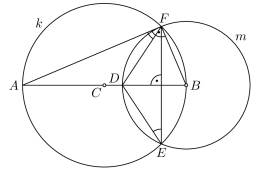Did you find an error or inaccuracy? Feel free to write us. Thank you!Tips to related online calculators
Pythagorean theorem is the base for the right triangle calculator.
Do you want to convert length units?

#### You need to know the following knowledge to solve this word math problem:

We encourage you to watch this tutorial video on this math problem:

## Related math problems and questions:

• Circle tangentIt is given to a circle with the center S and radius 3.5 cm. Distance from the center to line p is 6 cm. Construct a circle tangent n which is perpendicular to the line p.
• TangentsTo circle with a radius of 41 cm from the point R guided two tangents. The distance of both points of contact is 16 cm. Calculate the distance from point R and circle centre.
• Chord 2Point A has a distance of 13 cm from the center of the circle with a radius r = 5 cm. Calculate the length of the chord connecting the points T1 and T2 of contact of tangents led from point A to the circle.
• Two chordsIn a circle with radius r = 26 cm two parallel chords are drawn. One chord has a length t1 = 48 cm and the second has a length t2 = 20 cm, with the center lying between them. Calculate the distance of two chords.
• Tangent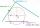What distance is the tangent t of the circle (S, 4 cm) and the chord of this circle, which is 6 cm long and parallel to the tangent t?
• CirclesIn the circle with a radius 7.5 cm are constructed two parallel chord whose lengths are 9 cm and 12 cm. Calculate the distance of these chords (if there are two possible solutions write both).
• Circle in rhombus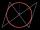In the rhombus is an inscribed circle. Contact points of touch divide the sides to parts of length 19 cm and 6 cm. Calculate the circle area.
• Circle chordCalculate the length of the chord of the circle with radius r = 10 cm, length of which is equal to the distance from the center of the circle.
• NonagonCalculate the area and perimeter of a regular nonagon if its radius of the inscribed circle is r = 10cm
• Right 24Right isosceles triangle has an altitude x drawn from the right angle to the hypotenuse dividing it into 2 unequal segments. The length of one segment is 5 cm. What is the area of the triangle? Thank you.
• Right isosceles triangleRight isosceles triangle has an altitude x drawn from the right angle to the hypotenuse dividing it into 2 equal segments. The length of one segment is 5 cm. What is the area of the triangle?
• Circle describedThe radius of the circle described to the right triangle with 6 cm long leg is 5 cm. Calculate the circumference of this triangle.
• Tangent 3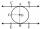In a circle with centre O radius is 4√5 cm. EC is the tangent to the circle at point D. Segment AB IS THE DIAMETER of given circle. POINT A is joined with POINT E and POINT B is joined with POINT C. Find DC if BC IS 8cm.
• Chord 4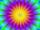I need to calculate the circumference of a circle, I know the chord length c=22 cm and the distance from the center d=29 cm chord to the circle.
• Center of line segmentCalculate the distance of the point X [1,3] from the center of the line segment x = 2-6t, y = 1-4t ; t is from interval <0,1>.
• RT triangle and heightCalculate the remaining sides of the right triangle if we know side b = 4 cm long and height to side c h = 2.4 cm.
• Chord ABWhat is the chord AB's length if its distance from the center S of the circle k(S, 92 cm) is 10 cm?